### Reference: Analyzing chart data

This topic contains reference information about the methods Quattro Pro provides for analyzing chart data.

The moving average method smoothes fluctuating data points by plotting progressive averages. This helps eliminate cyclic, seasonal, and fluctuating patterns and can accentuate a trend’s direction and substantiate trend reversals. Line and area charts are most effective for displaying moving averages.

Starting with the first point in the series, Quattro Pro calculates and plots the average for a specified previous number of points, called a period. At each following point, Quattro Pro maintains the specified period: it drops the oldest value (the one farthest from the new point) so the number of points averaged is always the specified period. Quattro Pro then calculates and plots the new average, and continues in this way. For example, if daily sales vary widely, you can determine a general trend by smoothing data points with the period set at three points to be averaged. In this case, each day’s sales would be averaged with the previous two so that each point is tempered by previous points and data is smoothed to show a general trend.

The moving average with a period of three provides a smoother line through the daily data. A moving average with a period of nine provides an even smoother effect, although it is less true to the daily totals.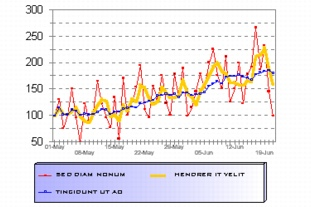This is an example of a chart that includes two moving averages with daily data.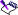• Moving averages can only be determined for chart series that contain three or more data points.

Aggregation combines multiple data points and plots them as a single point that can be the sum, average, standard deviation, minimum, or maximum of the data. Plotting aggregates reveals relationships not immediately apparent in the spreadsheet, such as weekly averages for information recorded daily.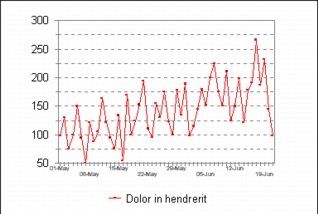This is an example of daily sales data aggregated so that each point represents a weekly sales average.

In the example above, series period is set to days, aggregation period is set to weeks, and function is set to AVG. It helps to think of the aggregation commands as useful standards for simple periodic aggregation. For example, when you specify days for the series period, months for the aggregation period, and AVG for function, Quattro Pro will average 30 data points, plot the average, average the next 30 points, plot the average, and so on.

Line charts work well with aggregation because they easily show trends. Bar charts are also impressive when plotting aggregated data over time, although trends are not as easy to see when you chart multiple series in the same chart.

You can modify aggregate charts for the following options: series period, aggregation period, and function. Since you will often aggregate a series according to a time period, the aggregation commands are based on one day representing a period of one. For the purposes of aggregation, Quattro Pro uses the following standards.

 Time Period Equals Week 7 days Month 30 days = 4 weeks Quarter 90 days = 12 weeks = 3 months Year 360 days = 51 weeks = 12 months = 4 quarters

Regression analysis is a mathematical method that shows relationships between multiple variables, so it is often used to predict values of one variable by taking into account the values of the others. In Quattro Pro, a linear fit series generates a regression line that best fits the data. Linear fit is useful for showing a general trend among fluctuating points.

Linear fit calculates and plots regression information in a line, even if the data does not have a general trend.

The linear fit trendline is of the form where x and y are the values of the two variables for which a relationship is being developed. Such a model would be used when one assumes a linear relationship between the dependent variable, Y, and the independent variable, X. The estimated regression coefficients are determined as follows: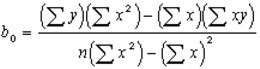The exponential fit method generates a curve to fit data that increases or decreases geometrically (through multiplication rather than addition). For this feature to work, all values in the series must be greater than zero.

If you specify an area larger than the number of existing data points, additional values are projected, based on the plotted curve.

In any exponential fit charts, you can display different data series and data relationships. For example, you can compare weekly maximum sales totals with weekly sales averages.

The original and transformed model equations for the exponential fit are:

Original equation of fit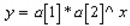Transformed equation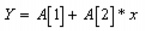where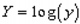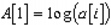Once the coefficients A and A are found using least squares techniques, an exponential transformation is applied in order to maintain the integrity of the model of interest.

#### Standard deviation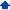The standard deviation fit plots solid horizontal lines at values corresponding to the mean plus/minus the standard deviation for the data set plotted in the chart. Doing so enables the user to get a visual interpretation both of outlying observations that may be of particular interest for future investigation, as well as the proximity of other data points to the overall average.

The common log fit summarizes trends using the following model equations:

Original equation of fit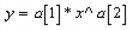Transformed equation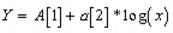where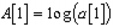Coefficients are determined using least squares techniques and inverse transformations are then applied where necessary in order to maintain the integrity of the models of interest.

When performing a common log fit, values may be either positive or negative.

Coefficients are determined using least squares techniques and inverse transformations are then applied where necessary in order to maintain the integrity of the models of interest.

When performing a natural log fit, all values in the chart series must be greater than zero.

A polynomial trendline can be used to model fluctuation in a given data series. Depending on the manner in which data changes over time, you can use polynomials of different orders to adequately fit the data; an order of 2 is useful in instances where there is only one maximum or minimum value in an otherwise parabolically shaped data series, whereas polynomials of higher order can model data with more “hills and valleys.” This form of trendline is useful in cases where the relationship between two variables is thought to be curvilinear rather than linear.

The model being fit under this scenario is given by: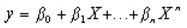where.

Thus, the feature can fit polynomials up to and including an order of 4. Coefficients for the polynomial fit are determined using least squares techniques.

#### Calculating error amountsError amounts Fixed amount The error amount is the value that you specify. Percentage The error amount is calculated based on the percentage you specify of the initial data point. Standard deviation The error amount is calculated based on the standard deviation formula.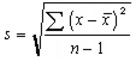Standard error The error amount is calculated based on the standard error formula.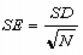Custom The error amount represents the negative and positive values you specify.